Courses

# Wave Guides (Part - 2) Electrical Engineering (EE) Notes | EduRev

## Electromagnetic Theory

Created by: Machine Experts

## Electrical Engineering (EE) : Wave Guides (Part - 2) Electrical Engineering (EE) Notes | EduRev

The document Wave Guides (Part - 2) Electrical Engineering (EE) Notes | EduRev is a part of the Electrical Engineering (EE) Course Electromagnetic Theory.
All you need of Electrical Engineering (EE) at this link: Electrical Engineering (EE)

Rectangular Waveguide

In the last lecture, we have coonsidered the case of guided wave between a pair of infinite conducting planes. In this lecture, we consdier a rectangular wave guide which consists of a hollow pipe of infinite extent but of rectangular cross section of dimension α x b. The long direction will be taken to be the z direction.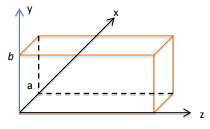Unlike in the previous case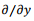is not zero in this case. However, as the propagation direction is along the z direction, we have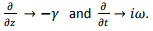We can write the Maxwell’s curlequations as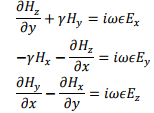The second set of equations are obtained from the Faraday’s law, (these can be written down from above by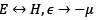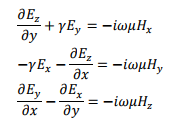As in the case of parallel plate waveguides, we can express all the field quantities in terms of the derivatives of Eand Hz . For instance, we have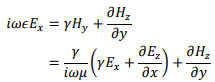which gives,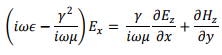so that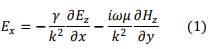where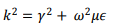The other components can be similarly written down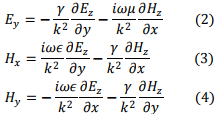As before, we will look into the TE mode in detail. Since Ez = 0, we need to solve for Hz from the Helmholtz equation,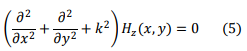Remember that the complete solution is obtained by multiplying with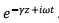We solve equation (5) using the technique of separation of variables which we came across earlier. Let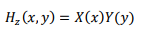Substituting this in (5) and dividing by XY throughout, we get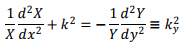where Ky is a constant. We further define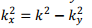We now have two second order equations,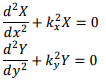The solutions of these equations are well known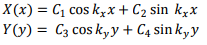This gives,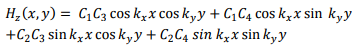The boundary conditions that must be satisfied to determine the constants is the vanishing of the tangential component of the electric field on the plates. In this case, we have   two pairs of plates. The tangential direction on the plates at x= 0 and x = α is the y direction, so that the y component of the electric field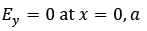Likewise, on the plates at y = 0 and y = b,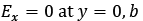We need first to evaluate Ex and Ey using equations (1) and (2) and then substitute the boundary conditions. Since Ez = 0, we can write eqn. (1) and (2) as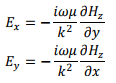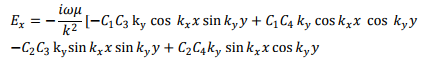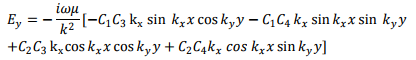Since Ey = 0 at x = 0, we must have C2 = 0 and then we get,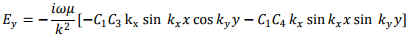Further, since Ex = 0 at y=0, we have C= 0. Combining these, we get, on defining a constant C = C1C3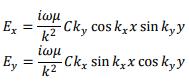And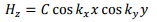We still have the boundary conditions, Ex= 0 at y = b and Ey = 0 at x = α to be satisfied. The former gives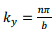while the latter gives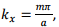where m and n are integers. Thus we have,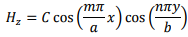and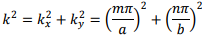However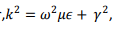so that,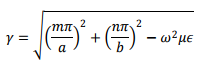For propagation to take place, γ must be imaginary, so that the cutoff frequency below which propagation does not take place is given by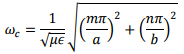The minimum cutoff is for TE1,0 (or TE0,1 ) mode which are known as dominant mode. For these modes Ex (or Ey ) is zero.

TM Modes

We will not be deriving the equations for the TM modes for which Hz = 0 . In this case, the solution for Ez , becomes,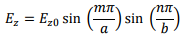As the solution is in terms of product of sine functions, neither m nor n can be zero in this case. This is why the lowest TE mode is the dominant mode

For propagating solutions, we have,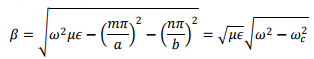where,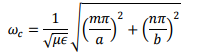We have,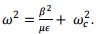Differentiating both sides, we have,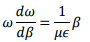The group velocity of the wave is given by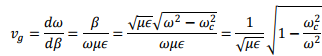which is less than the speed of light. The phase velocity, however, is given by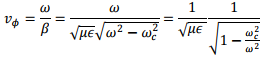It may be noted that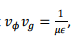which in vacuum equal the square velocity of light.

For propagating TE mode, we have, from (1) and (4) using Ez = 0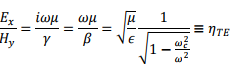where ηTE is the characteristic impedance for the TE mode. It is seen that the characteristic impedance is resistive. Likewise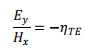Power Transmission

We have seen that in the propagating mode, the intrinsic impedance is resistive, indicating that there will be average flow of power. The Poynting vector for TE mode is given by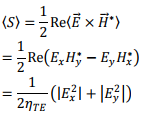Substituting the expressions for the field components, the average power flow through the x y plane is given by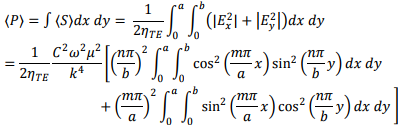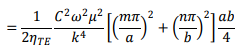Impossibility of TEM mode in Rectangular waveguides

We have seen that in a parallel plate waveguide, a TEM mode for which both the electric and magnetic fields are perpendicular to the direction of propagation, exists. This, however is not true of rectangular wave guide, or for that matter for any hollow conductor wave guide without an inner conductor.

We know that lines of H are closed loops. Since there is no z component of the magnetic field, such loops must lie in the x-y plane. However, a loop in the x-y plane, according to Ampere’s law, implies an axial current. If there is no inner conductor, there cannot be a real current. The only other possibility then is a displacement current. However, an axial displacement current requires an axial component of the electric field, which is zero for the TEM mode. Thus TEM mode cannot exist in a hollow conductor. (for the parallel plate waveguides, this restriction does not apply as the field lines close at infinity.)

Resonating Cavity

In a rectangular waveguide we had a hollow tube with four sides closed and a propagation direction which was infinitely long. We wil now close the third side and consider electromagnetic wave trapped inside a rectangular parallopiped of dimension α × b × d with walls being made of perfector conductor. (The third dimension is taken as d so as not to confuse with the speed of light c).

Resonanting cavities are useful for storing electromagnetic energy just as LC circuit does but the former has an advantage in being less lossy and having a frequency range much higher, above 100 MHz.

The Helmholtz equation for any of the components Eα of the electric field can be written as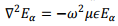We write this in Cartesian and use the technique of separation of variables,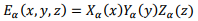Introducing this into the equation and dividing by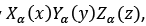, we get,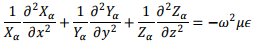Since each of the three terms on the right is a function of an independent variable, while the right hand side is a constant, we must have each of the three terms equaling a constant such that the three constants add up to the constant on the right. Let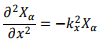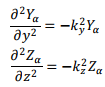such that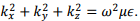As each of the equations has a solution in terms of linear combination of sine and cosine functions, we write the solution for the electric field as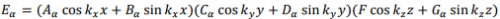Tha tangential component of above must vanish at the metal boundary. This implies,

1. At x= 0 and at x = α , Ey and Ez = 0 for all values of y,z
2. At y = 0 and y = b, Ex and Ez = 0 for all values of x,z
3. At z = 0 and z=d, Ex and Ey = 0 for all values of x, y

Let us consider Ex which must be zero at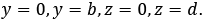. This is posible if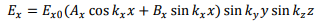with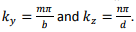Here m and n are non-zero integers.

In a similar way, we have,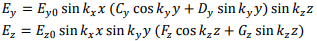with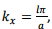with ℓ being non-zerointeger.

We now use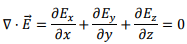This relation must be satisfied for all values of x, y, z within the cavity. This requires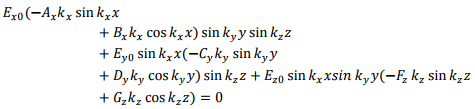Let us choose some special points and try to satisfy this equation. Let x = 0, y, z arbitrary,

This requires Bx = 0. Likewise, takingy = 0, x, z arbitrary, we require Dy = 0 and finally, z = 0,

x, yarbitrary gives Gz = 0.

With these our solutions for the components of the electric field becomes,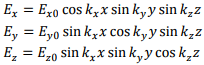where we have redefined our constants Ex0, Ey0 and Ez0.

We also have,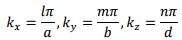the integers ℓ, m, n cannot be simultaneously zero for then the field will identically vanish. Note further that since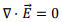must be satisfied everywhere within the cavity, we must have, calculating the divergence explicitly from the above expressions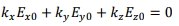This requires that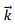is perpendicular to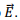One can see that the modes within a cavity can exist only with prescribed “resonant” frequency corresponding to the set of integers ℓ, m, n,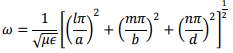Though there is nothing like a propagation direction here, one can take the longest dimension (say d) to be the propagation direction.

We can have modes like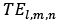corresponding to the set l, m, n for which Ez = 0. For this set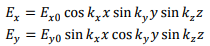with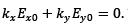The magnetic field components can be obtained from the Faraday’s law,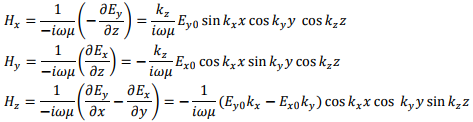Tutorial Assignment

1. A rectangular waveguide has dimensions 8 cm x 4 cm. Determine all the modes that can propagate when the operating frequency is (a) 1 GHz, (b) 3 GHz and (c) 8 GHz.
2. Two signals, one of frequency 10 GHz and the other of 12 GHz are simultaneously launched in an air filled rectangular waveguide of dimension 2 cm x 1 cm. Find the time interval between the arrival of the signals at a distance of 10 m from the common place of their launch.
3. What should be the third dimension of a cavity having a length of 1 cm x 1 cm which can operate in a TE103 mode at 24 GHZ?

Solutions to Tutorial Assignments

1. The cutoff frequency for (m,n) mode is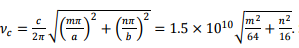. Some of the lowest cutoffs are as under (in GHz) :

 m n Cutoff frequency (GHz) 0 1 3.75 0 2 7.5 0 3 11.25 1 0 1.875 1 1 4.192 1 2 7.731 1 3 11.405 2 0 3.75 2 1 5.30 2 2 8.39 3 0 5.625 3 1 6.76 3 2 9.375 4 0 7.5 4 1 8.38 5 0 9.375

At 1 GHz, no propagation takes place. At 3 GHz only TE10 mode propagates (recall no TM mode is possible when either of the indices is zero.) At 8 GHz, we have TE01, TE02, TE10, TE11, TE12, TE20, TE21, TE30, TE31, TE40, TM11, TM12, TM21 and TM31, i.e. a total of 14 modes propagating.

2. The cutoff frequency is given by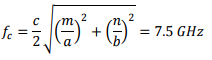The propagation of the wave is given by the group velocity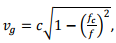which is 1.98x 10and 2.07x10m/s respectively for f=10 GHz and 12 GHz respectively.           Thus the difference in speed is 9x 106 m/s, resulting in a time difference of approximately 10-5 s in travelling 10m.

3. The operating frequency is given by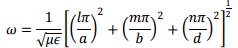Substitutingl = 1, m = 0, n = 3, we get d = 2.4 cm

Self Assessment Questions

1. A rectangular, air filled waveguide has dimension 2 cm x 1 cm. For what range of frequencies, there is a “single mode” operation in the guide?
2. The cutoff frequency for a TE10 mode in an air filled waveguide is 1.875 GHz. What would be the cutoff frequency of this mode if the guide were to be filled with a dielectric of permittivity 9∈0?
3. In an air filled waveguide of dimension 2 cm x 2 cm, the x component of the electric field is given by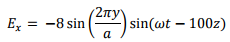Identify the propagating mode, determine the frequency of operation and find Hz and Ez .

Solutions to Self Assessment Questions

1. The cutoff frequency for (m,n) mode is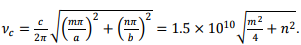Substituting values, the cutoff for (1,0) mode is 7.5 GHz and for (2,0) is 15 GHz. The frequency for (0,1) is also 15 GHz. All other modes have higher cut off. Thus in order that only one mode propagates, the operating frequency should be in the range 7.5 < ν < 15 GHz. In this range, only TE10 mode operates. Recall that there is no TM mode with either of the indices being zero.
2. The cutoff frequency is proportional to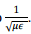Thus the frequency would be reduced by a factor of 3 making it 625 MHz.
3. Since one of the standing wave factor is missing, one of the mode indices is zero. Thus it is a TE mode. The electric field of TEmn mode is given by (with a=b)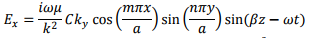Thus the mode is TE02 mode. From the relation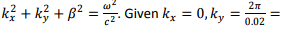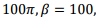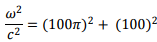the operating                   frequency is 15.74 GHz.

Since kx = 0, Ey = 0 . Further, by definition for TE mode Ez= 0.

Here we have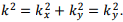The ratio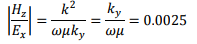so that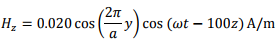,

,

,

,

,

,

,

,

,

,

,

,

,

,

,

,

,

,

,

,

,

;